Trie 树那节我们讲过，利用 Trie 树，可以实现搜索引擎的关键词提示功能，这样可以节省用户输入搜索关键词的时间。实际上，搜索引擎在用户体验方面的优化还有很多，比如你可能经常会用的拼写纠错功能。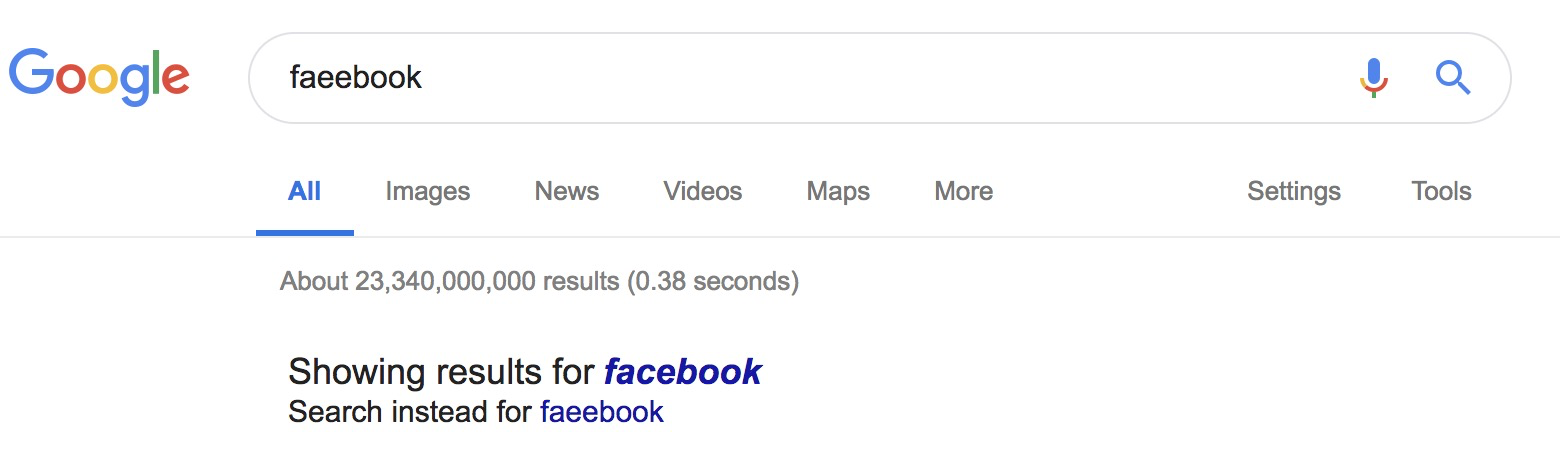## 如何量化两个字符串的相似度？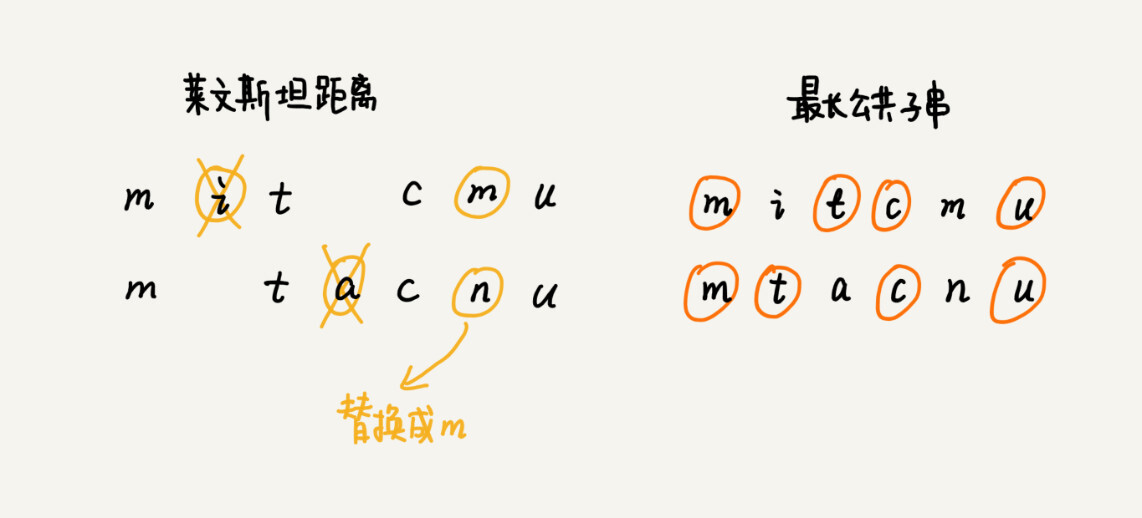## 如何编程计算莱文斯坦距离？

```private char[] a = "mitcmu".toCharArray();
private char[] b = "mtacnu".toCharArray();
private int n = 6;
private int m = 6;
private int minDist = Integer.MAX_VALUE; // 存储结果
// 调用方式 lwstBT(0, 0, 0);
public lwstBT(int i, int j, int edist) {
if (i == n || j == m) {
if (i < n) edist += (n-i);
if (j < m) edist += (m - j);
if (edist < minDist) minDist = edist;
return;
}
if (a[i] == b[j]) { // 两个字符匹配
lwstBT(i+1, j+1, edist);
} else { // 两个字符不匹配
lwstBT(i + 1, j, edist + 1); // 删除a[i]或者b[j]前添加一个字符
lwstBT(i, j + 1, edist + 1); // 删除b[j]或者a[i]前添加一个字符
lwstBT(i + 1, j + 1, edist + 1); // 将a[i]和b[j]替换为相同字符
}
}```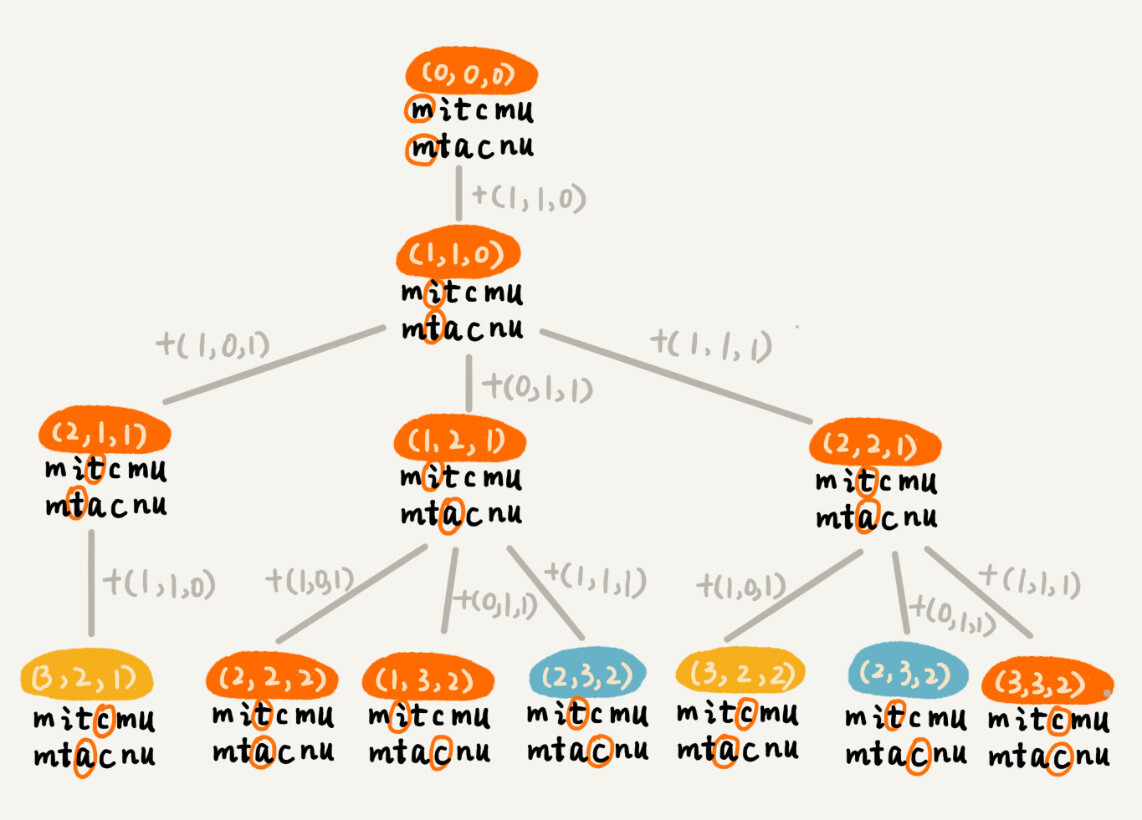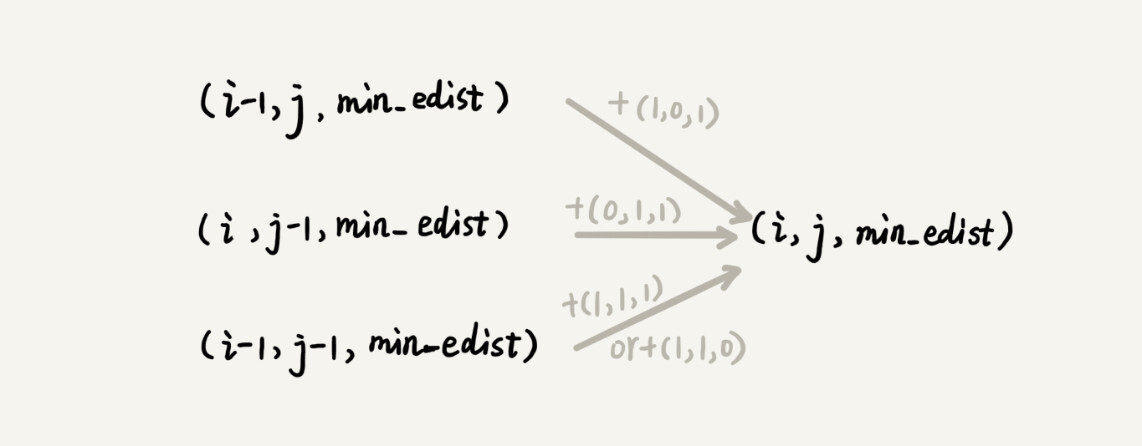```如果：a[i]!=b[j]，那么：min_edist(i, j)就等于：
min(min_edist(i-1,j)+1, min_edist(i,j-1)+1, min_edist(i-1,j-1)+1)

min(min_edist(i-1,j)+1, min_edist(i,j-1)+1，min_edist(i-1,j-1))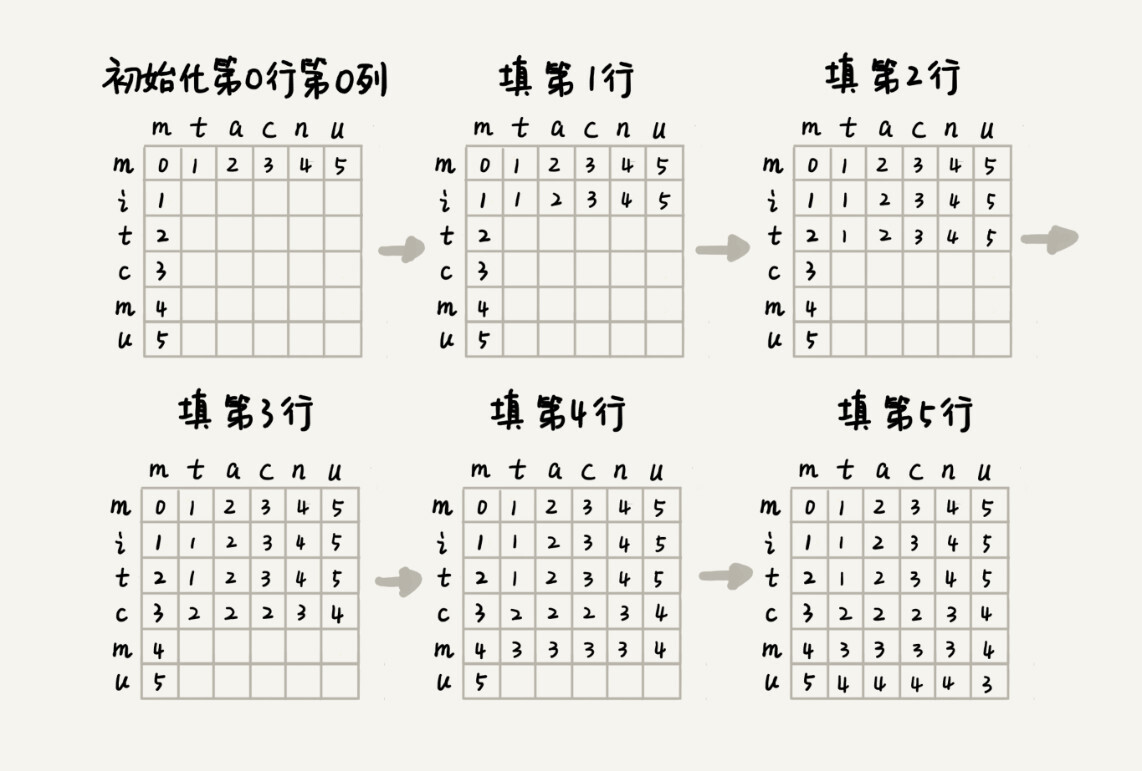```public int lwstDP(char[] a, int n, char[] b, int m) {
int[][] minDist = new int[n][m];
for (int j = 0; j < m; ++j) { // 初始化第0行:a[0..0]与b[0..j]的编辑距离
if (a == b[j]) minDist[j] = j;
else if (j != 0) minDist[j] = minDist[j-1]+1;
else minDist[j] = 1;
}
for (int i = 0; i < n; ++i) { // 初始化第0列:a[0..i]与b[0..0]的编辑距离
if (a[i] == b) minDist[i] = i;
else if (i != 0) minDist[i] = minDist[i-1]+1;
else minDist[i] = 1;
}
for (int i = 1; i < n; ++i) { // 按行填表
for (int j = 1; j < m; ++j) {
if (a[i] == b[j]) minDist[i][j] = min(
minDist[i-1][j]+1, minDist[i][j-1]+1, minDist[i-1][j-1]);
else minDist[i][j] = min(
minDist[i-1][j]+1, minDist[i][j-1]+1, minDist[i-1][j-1]+1);
}
}
return minDist[n-1][m-1];
}

private int min(int x, int y, int z) {
int minv = Integer.MAX_VALUE;
if (x < minv) minv = x;
if (y < minv) minv = y;
if (z < minv) minv = z;
return minv;
}```

## 如何编程计算最长公共子串长度？

(i-1, j-1, max_lcs)，其中 max_lcs 表示 a[0...i-1]和 b[0...j-1]的最长公共子串长度；

(i-1, j, max_lcs)，其中 max_lcs 表示 a[0...i-1]和 b[0...j]的最长公共子串长度；

(i, j-1, max_lcs)，其中 max_lcs 表示 a[0...i]和 b[0...j-1]的最长公共子串长度。

```如果：a[i]==b[j]，那么：max_lcs(i, j)就等于：
max(max_lcs(i-1,j-1)+1, max_lcs(i-1, j), max_lcs(i, j-1))；

max(max_lcs(i-1,j-1), max_lcs(i-1, j), max_lcs(i, j-1))；

```public int lcs(char[] a, int n, char[] b, int m) {
int[][] maxlcs = new int[n][m];
for (int j = 0; j < m; ++j) {//初始化第0行：a[0..0]与b[0..j]的maxlcs
if (a == b[j]) maxlcs[j] = 1;
else if (j != 0) maxlcs[j] = maxlcs[j-1];
else maxlcs[j] = 0;
}
for (int i = 0; i < n; ++i) {//初始化第0列：a[0..i]与b[0..0]的maxlcs
if (a[i] == b) maxlcs[i] = 1;
else if (i != 0) maxlcs[i] = maxlcs[i-1];
else maxlcs[i] = 0;
}
for (int i = 1; i < n; ++i) { // 填表
for (int j = 1; j < m; ++j) {
if (a[i] == b[j]) maxlcs[i][j] = max(
maxlcs[i-1][j], maxlcs[i][j-1], maxlcs[i-1][j-1]+1);
else maxlcs[i][j] = max(
maxlcs[i-1][j], maxlcs[i][j-1], maxlcs[i-1][j-1]);
}
}
return maxlcs[n-1][m-1];
}

private int max(int x, int y, int z) {
int maxv = Integer.MIN_VALUE;
if (x > maxv) maxv = x;
if (y > maxv) maxv = y;
if (z > maxv) maxv = z;
return maxv;
}```